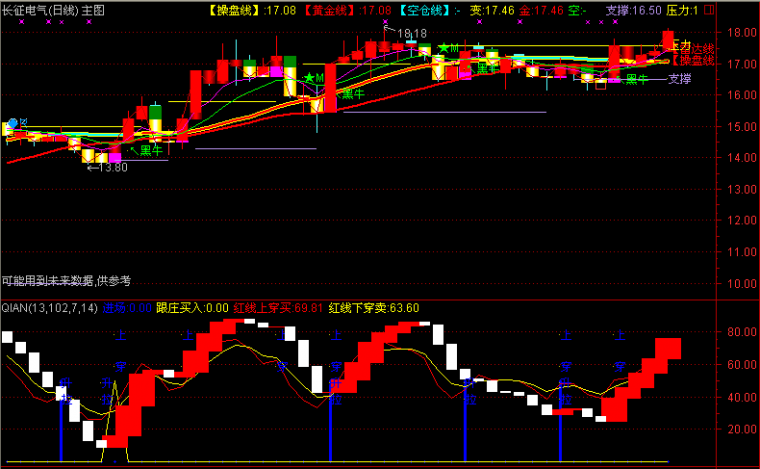﻿ 拉升在即_买入大涨-大智慧公式 －程序化交易（CXH99.COM）

# 拉升在即_买入大涨[大智慧公式]

A赋值:收盘价>1日前的收盘价
A1赋值:统计N日中满足A的天数=N
B赋值:成交量(手)>1日前的成交量(手)
B1赋值:统计N日中满足B的天数=N
(收盘价-开盘价)/2日前的收盘价*100<5 AND A1 AND B1
Z1赋值:收盘价的13日简单移动平均
ZX赋值:13/2+1日前的Z1
A1赋值:最高价的21日[2日权重]移动平均*1.05
A2赋值:最低价的21日[2日权重]移动平均*0.95
A3赋值:最低价的5日[1日权重]移动平均
A4赋值:收盘价的2日[1日权重]移动平均

input: n(3);
a:=c>ref(c,1);
a1:=count(a,n)=n;
b:=v>ref(v,1);
b1:=count(b,n)=n;
(c-o)/ref(c,2)*100<5 and a1 and b1;{买入大涨}
Z1:=MA(C,13);
ZX:=REF(Z1,13/2+1);
A1:=SMA(HIGH,21,2)*1.05;
A2:=SMA(LOW,21,2)*0.95;
A3:=SMA(LOW,5,1);
A4:=SMA(CLOSE,2,1);

XG:IF(大涨>A2 OR 买入>A3 AND CROSS(C,ZX) AND VOL>0,10,0);(注：由于人数限制，QQ或微信请选择方便的一个联系我们就行，加好友时请简单备注下您的需求，否则无法通过。谢谢您！)

【字体： 】【打印文章】【查看评论

没有相关内容